# Test: Basic BJT Circuits

## 20 Questions MCQ Test GATE ECE (Electronics) 2023 Mock Test Series | Test: Basic BJT Circuits

Description
Attempt Test: Basic BJT Circuits | 20 questions in 60 minutes | Mock test for Electronics and Communication Engineering (ECE) preparation | Free important questions MCQ to study GATE ECE (Electronics) 2023 Mock Test Series for Electronics and Communication Engineering (ECE) Exam | Download free PDF with solutions
QUESTION: 1

Solution:
QUESTION: 2

### For the transistor in circuit shown in fig. β = 200. Determine the value of VC for given value of VB in question.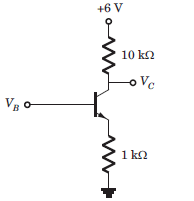If VB = 1V

Solution: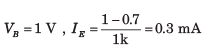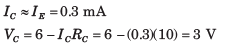QUESTION: 3

### For the transistor in circuit shown in fig. β = 200. Determine the value of IE and IC for given value of VB in question.If VB = 2V

Solution: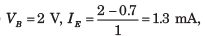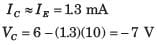Transistor is in stauration. The saturation voltage
VCE = 0.2V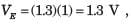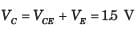QUESTION: 4

The transistor in circuit shown in fig. has β = 200. Determine the value of voltage Vo for given value of VBB.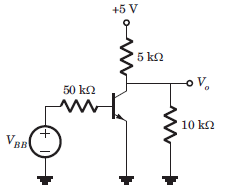If VBB = 0

Solution:

VBB = 0, Transistor is in cutoff region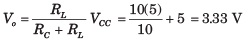QUESTION: 5

The transistor in circuit shown in fig. has β = 200. Determine the value of voltage Vo for given value of VBB.If VBB = 1V

Solution: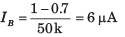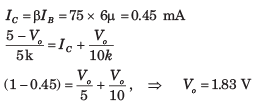QUESTION: 6

The transistor in circuit shown in fig. has β = 200. Determine the value of voltage Vo for given value of VBB.If VBB = 2V

Solution: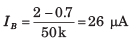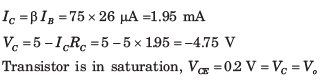QUESTION: 7

The transistor shown in the circuit of fig. has β = 150. Determine Vo for given value of
IQ in question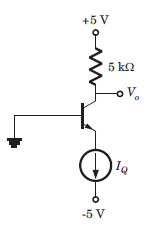If IQ = 0.1 mA

Solution:

I= 0.1 mA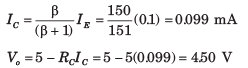QUESTION: 8

The transistor shown in the circuit of fig. has β = 150. Determine Vo for given value of  IQ in questionIf IQ = 0.5 mA

Solution:

IE = IQ = 0.5 mA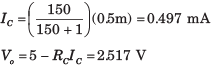QUESTION: 9

The transistor shown in the circuit of fig. has β = 150. Determine Vo for given value of  IQ in questionIf IQ = 2mA

Solution:

Transistor is in saturation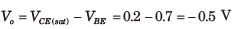QUESTION: 10

For the circuit in fig. VB = V and  β = 50. The value of VB is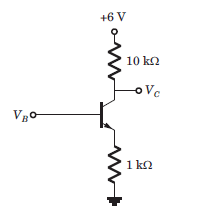Solution: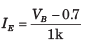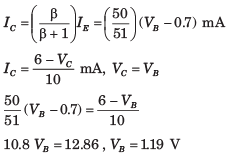QUESTION: 11

For the circuit shown in fig. VCB = 0.5 V and β =100. The value of IQ is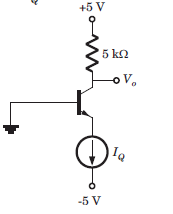Solution: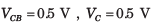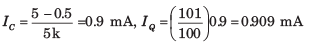QUESTION: 12

For the circuit shown in fig. the emitter voltage is VE = 2 V. The value of α is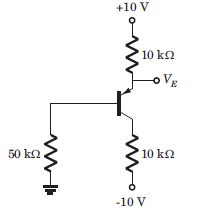Solution: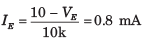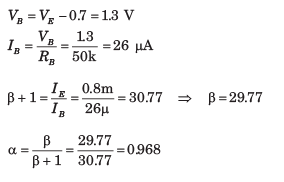QUESTION: 13

For the transistor in fig. β = 50. The value of voltage VEC  is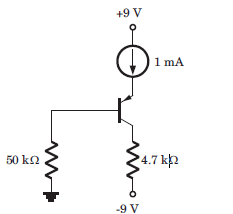Solution: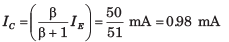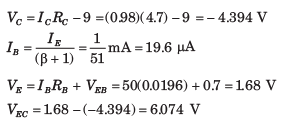QUESTION: 14

The current gain of the transistor shown in the circuit of fig. is β = 125. The Q-point values (ICQ , VCEQ ) are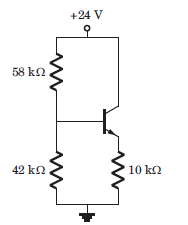Solution: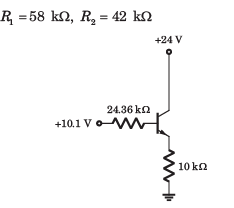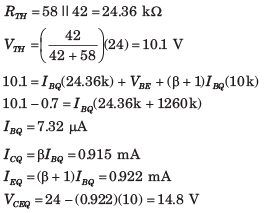QUESTION: 15

For the circuit shown in fig. , let β = 75. The Q-point (ICQ , VCEQ ) is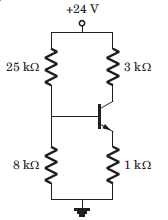Solution: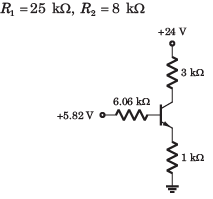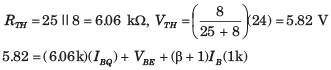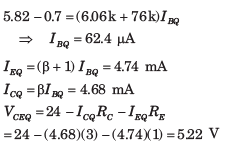QUESTION: 16

The current gain of the transistor shown in the circuit of fig. is β = 100. The values of Q-point (ICQ , VCEQ ) is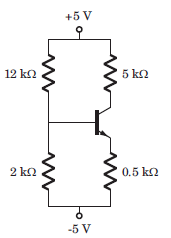Solution:

R1 =12 Ω, R2 = 2 Ω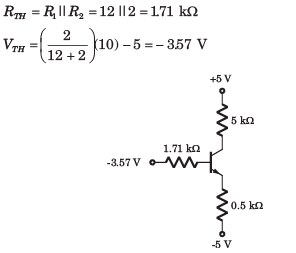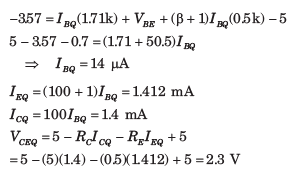QUESTION: 17

For the circuit in fig. , let β = 60. The value of VECQ  is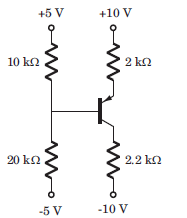Solution: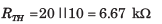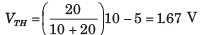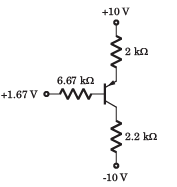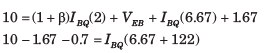QUESTION: 18

All transistor in the N output mirror in fig. are matched with a finite gain β and early voltage VA = ∞. The expression for each load current is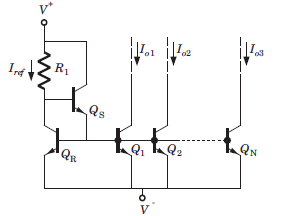Solution: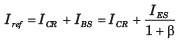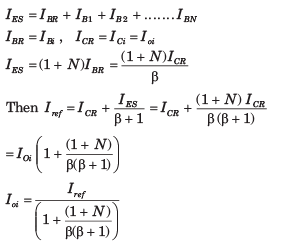QUESTION: 19

Consider the basic three transistor current source in fig. Assume all transistor are matched with finite gain and early voltage VA  = ∞.The expression for Io is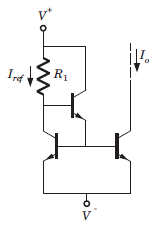Solution: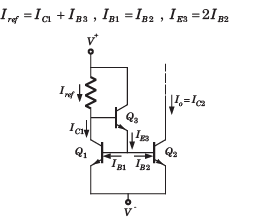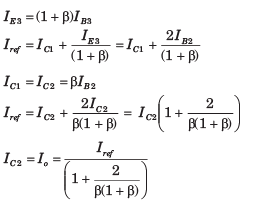QUESTION: 20

Consider the wilder current source of fig. Both of transistor are identical and β>>1 and VBE1 = 0.7 V. The value of resistance R1 and RE to produce Iref  = 1 mA and Io = 12 μA is (Vt = 0.026)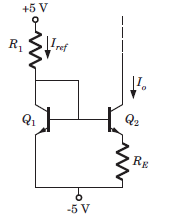Solution:

If β>>1 and transistor are identical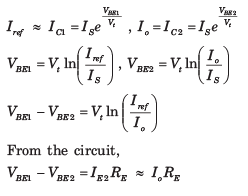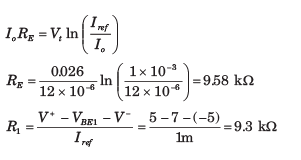Use Code STAYHOME200 and get INR 200 additional OFF Use Coupon Code